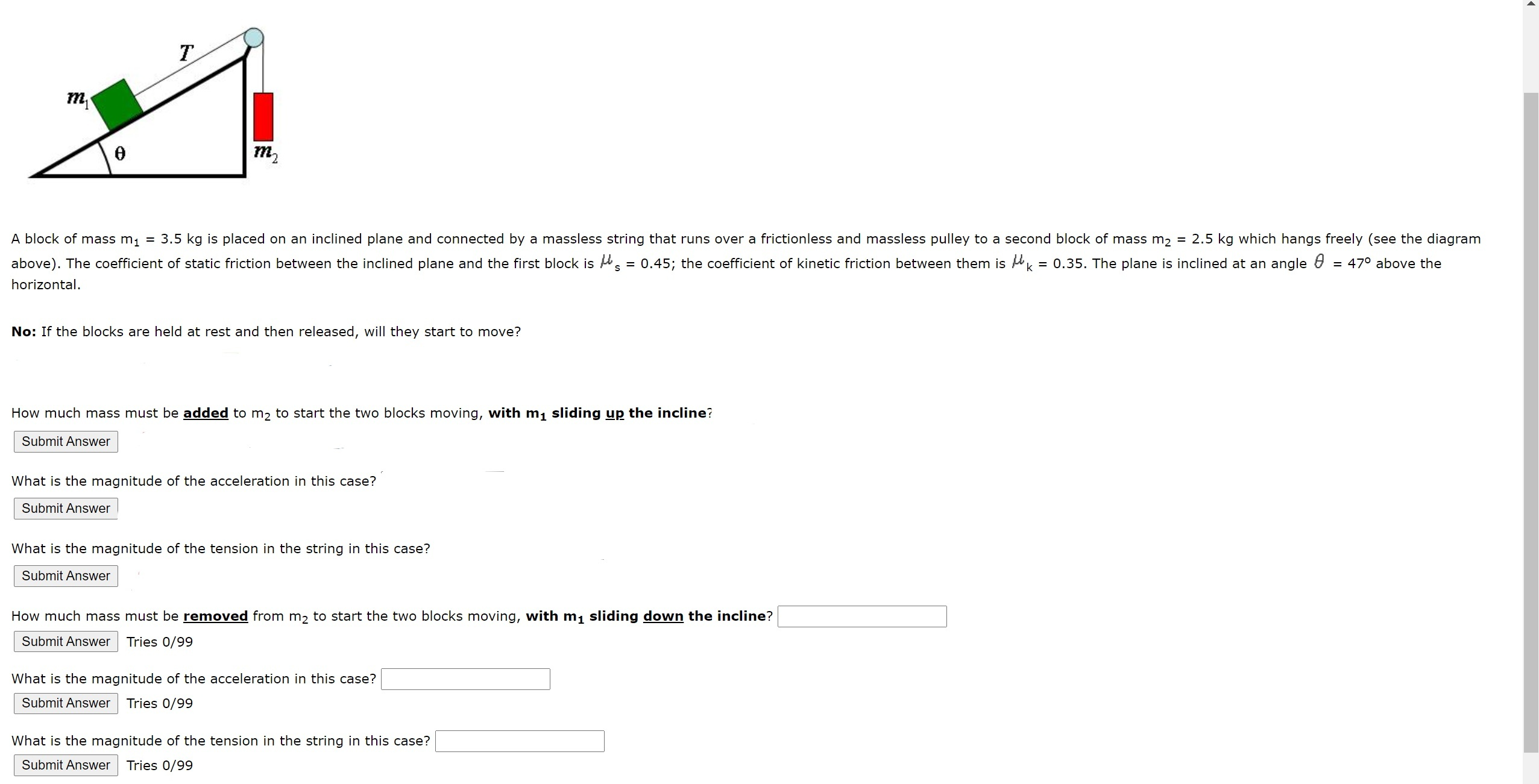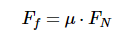## When Helping Means Winning contest

Ask a school-related question in the Help Zone and you could win one of three $500 scholarships. # Help Zone ### Student QuestionHi! I really really need help with this question, please. I am getting all the answers wrong and I do not know how to solve it. Physics{t c="richEditor.description.title"} {t c="richEditor.description.paragraphMenu"} {t c="richEditor.description.inlineMenu"} {t c="richEditor.description.embed"} ## Explanations (1) •Explanation from AlloprofExplanation from Alloprof This Explanation was submitted by a member of the Alloprof team. Hi NicePearl6852, Thank you for your question! The first step in this problem is to calculate the force of each block. Let's start with m2, as we can see it is only suspended in the air, therefore the only force applied on it is its weight. You can find that force with the equation F=mg, where g is the gravity (g=9,8 m/s2). m1 is a bit more complicated because it is on the inclined plane and there is friction. To find the force associated to its mass, you can use the following equation : $$F=mg\sin \theta$$ θ in this formula represents the inclination of the plane. The next step is to find the friction, here's the formula:μ in this formula is the coefficient of friction (static or kinetic). The use this formula you need the normal force, here's the formula needed to find it : F=mg\cos \theta$$Now you have everything to calculate the forces on the blocks, we can now look at the questions. 1) You need to find how heavy m2 must be to make m1 go up the plane, basically you need to find at what weight m2 will be stronger that the static friction to make m1 move. 2) Then as it moves, the friction becomes the kinectic friction, it becomes a weaker force. You can now find the acceleration because you have a resulting force, you'll need to use Newton's second law (F=ma) to find the acceleration. You need to find the sum of the forces on m1 and use F=ma. 3) Here are the force equations of m1 and m2: m1$$\sum F_{x}= T -m_{1}g\sin \theta - \mu_{k} m_{1}g\cos \theta = m_{1}a $$m2$$\sum F_{y}= T-m_{2}g =m_{2}a$\$

You need to find the value of T in these equations, it's the same in both, you can then validate your answer with the second equation.

For the last 3 questions, it is the same process as the three that I just explained, except that m1 is going down instead. I'll let you try it, I'm sure you are capable of doing it!!

Hope that helps!

Anthony B.